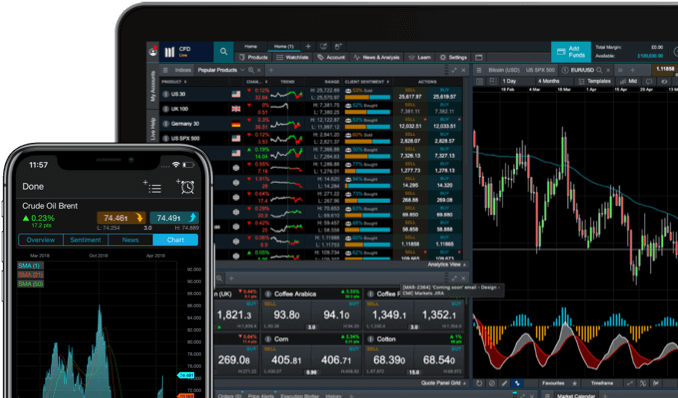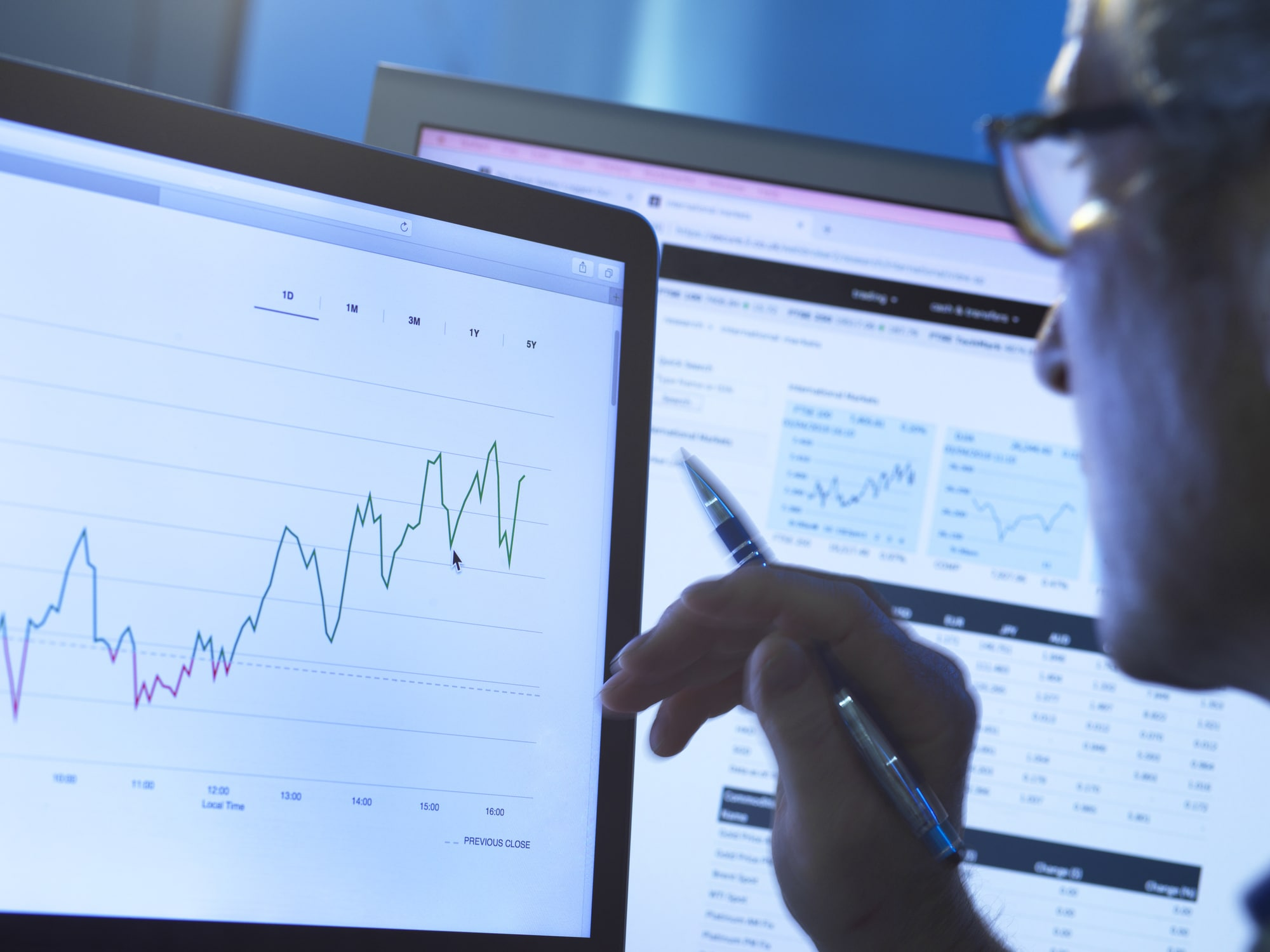Spread bets and CFDs are complex instruments and come with a high risk of losing money rapidly due to leverage. 77% of retail investor accounts lose money when spread betting and/or trading CFDs with this provider. You should consider whether you understand how spread bets and CFDs work and whether you can afford to take the high risk of losing your money.

# Standard deviation indicator

Standard deviation represents the technical foundation for effective online trading. Some of the greatest challenges that traders face is understanding the best time to enter the market and how to foresee possible trend reversals. With this in mind, one of the most useful concepts is standard deviation.

This volatility indicator​​ can have a great impact on investment strategies because it allows traders to better understand the up-and-down movements of the market that might prompt investors to enter a trade either too early or too late. Whereas more daring traders may be comfortable with an investment strategy that opts for above-average volatility, more conservative traders may not.

See inside our platform## What is standard deviation?

In online trading systems, standard deviation is a type of volatility indicators that is used to show and assess periods of volatility and to adjust the settings of other technical indicators, so that the system can react more rapidly to the signals it receives during high periods of high volatility. This includes when price trends change very quickly and investors must promptly open or close a trade.

In general, this indicator is used as a partial component with other indicators. For example, standard deviation can be used to determine the spread between the lower and upper Bollinger Bands​​.

## What does standard deviation show?

The standard deviation indicator measures market volatility and is used in statistics to describe the variability or dispersion of a set of data around the average. In technical analysis​​, it describes the price variability relative to a moving average (typically calculated at 20 days). The higher the standard deviation, the more unstable or volatile the market.

On the contrary, the lower the standard deviation, the more stable and steady the market; in other words, the price bars are very close to the moving average. It is a well-known fact, however, that market dynamics are characterised by an alternation of stable periods and peaks in activity.

## How to interpret standard deviation

The main interpretation of the standard deviation indicator is simple: if its value is too low – that is, if the market is completely stable – it is reasonable to expect a surge in activity in the near future. If, on the contrary, the indicator value is extremely high, the level of activity will likely slow down. Therefore, this information can alert traders to an imminent market entry opportunity.

Another use of the standard deviation in trading is to confirm upward or downward trends: as a rule, the market is less volatile during an upward trend, whereas during a downward trend or a market crash, you can witness high volatility due to an explosive volume of sale orders (also known as panic selling) initiated by investors.

## Standard deviation formula

In mathematical statistics, standard deviation is the square root of the variance. By calculating a standard deviation, we are simply translating the square root of the variance, using the following formula:

[σ (x) = √V (x)]

In turn, the variance in trading is the sum of the squared value obtained by subtracting the mean (x) from each value in the data set, divided by the number of values in said set.

## How to calculate standard deviation

Standard deviation can be calculated for assets within any market, including stocks, indices, commodities and forex.

As an example, consider Apple stock​​ (AAPL) over the past five years. The returns for Apple Inc shares were 10.03% in 2016, 46.11% in 2017, -6.79% in 2018, 86.16% in 2019, and 6.05% in 2020.

The average yield (m) in this five year period is 28.31%.

The return value for each year minus the mean is -18.28% in 2016, 17.8% in 2017, -35.1% in 2018, 57.85% in 2019 and -22.26% in 2020. You can then square the values to produce 334.15, 316.84, 1232.01, 3346.62 and 503.07.

The variance equals 1433.17, in which the squared values are added and divided by 4. Then, the square root of the variance is taken, which leaves us with a standard deviation of 37.35%.

Carry out technical analysis strategies

## Standard deviation and variance

Technically, standard deviation is the square root of the variance. In turn, the variance is the sum of the squared values obtained by subtracting the mean (x) from each value in the data set, divided by the number of the values in said set. Standard deviation measures the dispersion of the returns around the average return value. In other words, standard deviation is a measure of volatility. This is a key concept when it comes to financial risk because financial risk is seen as the possibility of returns dispersing around the expected value.

## How to use the standard deviation

Standard deviation can be used for three different purposes in trading:

• To identify outliers, because they can represent situations in which good opportunities might emerge (especially for those practicing scalping​​ and other advanced trading strategies​​)
• To choose points of entry based on trends: that is, understanding if some of the current prices have sufficiently deviated from the mean and could therefore return to their average value. If the trend is strong, investors could actually use the average price as the entry point.
• If prices instead indicate a narrow trading range and suddenly a strong standard deviation pushes prices away from the mean, investors can take advantage of the outlier values as an entry point.

## Why is volatility important for the standard deviation?

Volatility and standard deviation are closely related. Standard deviation is a statistical term that measures the amount of variability or dispersion around the average, and it is also a measure of volatility. Generally speaking, dispersion is the difference between the value and the average. The greater this dispersion or volatility, the greater the standard deviation. The lower this dispersion or variability, the lower the standard deviation.

### Example of volatility

Volatility can be calculated for any market: imagine, for example, that some investors are building a pension portfolio. Since they will be retiring in the coming years, they are actively looking for stocks with low volatility and stable returns.

Consider two companies:

Mitsubishi Motors Corporation has a stock beta​​ coefficient of 1.10, which makes it approximately as volatile as the S&P 500 index.

Peugeot S.A. has a beta coefficient of 1.78, making it significantly more volatile than the S&P 500 index.

## Standard deviation indicator MT4

We host the international MT4 platform, which offers multiple standard deviation indicators that have been created by users of the platform. Standard deviation is also often used with other MT4 indicators and add-ons, such as Bollinger Bands. These bands are plotted two standard deviations above and below a moving average. Movements beyond the bands are considered significant enough to be carefully evaluated. Register for an MT4 account now to get started.